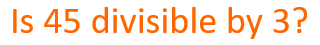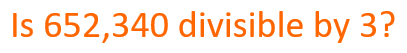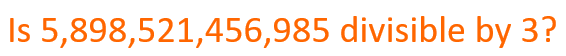Smartick is an advanced online program that teaches kids math and coding in only 15 min. a day

Jun07

# Follow the Divisibility Guidelines for 3

In the post for this week, I will explain the criteria for the divisibility of 3. When they ask you “Is this number divisible by 3?”  The most intuitive thing to do is to divide that number by 3, and if the remainder is zero then the number itself is divisible by 3.
For example:One way to know is to divide 45 by 3:

45/3=15

Since the remainder of the division is zero, we can say that 45 is divisible by 3.
But what if the number they ask us is too high?  Do we have to divide by 3 always?  The answer is NO. There is a much simpler way to calculate if a number is divisible by 3.  How? Very simple:
We add the digits of the number and if the result of the sum is a multiple of 3, then the number itself is divisible by 3.  If the result of the digit’s sums is a number that is not a multiple of 3, then the number is not divisible by 3. Easy, right?
Let’s try an example. As we already know that 45 is divisible by 3, we will see that the sum of its digits are a multiple of 3.

45 -> 4 + 5 = 9
9 is divisible by 3 therefore 45 is also divisible by 3.

Now let’s look at another example:We add up all the digits: 6 + 5 + 2 + 3 + 4 + 0 = 20
20 is not a multiple of 3, thus 652, 340 is not divisible by 3.

Want another example? Now an even longer one:5 + 8 + 9 + 8 + 5 + 2 + 1 + 4 + 5 + 6 + 9 + 8 + 5 = 75
7 + 5 = 12
12 is divisible by 3, thus 5,898,521,456,985 is divisible by 3.

As you can see, the divisibility of 3 is easy. Check out all of the different divisibility posts available on Smartick.